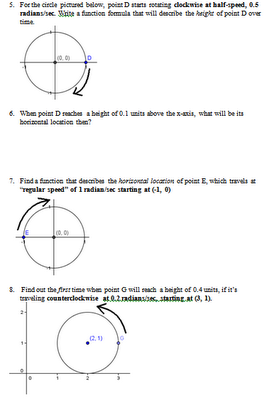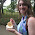## Friday, June 3, 2011

### Unit Circle and Wave Functions Project - Part 3

Just to round it out a bit, I thought I'd finish writing about how I leveraged my students' understanding of basic sine and cosine functions and their relationships to circles, in order to get at some basic solving of simple trig equations. It bothers me that in the textbook we'd see exercises like:
1 + 3*sin(5x) = 2 and kids would be expected to solve the equations without ever knowing what it means.

It's simple:
Let's say you've got a point C rotating around a circle of radius 3. The center of the circle is at (0, 1) and the point starts rotating at the normal "3 o'clock" position, (3, 1) in this case because the radius is 3 units. The rate of rotation is 5 radians/sec and the point rotates counterclockwise. At what times during the first cycle will this point reach a height of exactly 2 units above the x-axis?

A kid who understands how sine and cosine functions relate to circles should be able to write the function f(t) = 3*sin(5t) + 1 based on the above description. And then, they should be able to say, "I want to solve for time given the height is 2, so I'm going to plug 2 into the left hand side of the equation. Thus, 2 = 3*sin(5t) + 1." And then and only then should this kid find the two time values t that correspond to this y value! My kids can sketch a unit circle and articulate that we have two solutions (within the first full rotation) because there are two points along the circle with that same height. And then they can justify using a sketched circle why you find one radian solution, and then the other one is just pi minus that first solution (each of which you then divide by 5 to go from radians traveled to time it takes, if you are moving 5 radians/sec).

We can also illustrate the use of other simple trig equations such as [sin(x)]^2 + [cos(x)]^2 = 1 the same way. Instead of giving our kids cos(x) = -0.9 and asking them to solve algebraically for all other 5 trig values without knowing what it all means, why don't we instead say...

You have a circle whose radius is 1 and its center is (0, 0). Point C starts rotating around this circle counterclockwise at (1, 0) and moves at the regular speed of 1 radian/sec. Where (x, y) will Point C be after 5 seconds? When point C reaches a horizontal location of 0.9 units to the left of the y-axis, how high or low will it be? Find all 6 trig ratios at those points.

What kids should be able to do (with a bit of practice) is to go from something like f(x) = -2cos(0.5x) + 8 and g(x) = -2sin(0.5x) - 3 to drawing a circle of radius 2, centered at (8, -3), with its rotation starting at (6, -3) and moving at 0.5 radians/sec counterclockwise. From there, given a description of a certain height OR horizontal location, kids should be able to choose the appropriate equation, substitute in the appropriate value in the appropriate place, solve, and explain in a simple sentence what the numbers mean. And then, they should be able to take those waves and graph the wave functions while explaining the transformations that occurred to the waves as a result of the transformations inside the circle... thereby showing a full and flexible understanding of sines and cosines.

...I'm a newbie Precalc teacher, but I feel strongly we cannot be teaching kids how to solve trig equations -- even the basic ones! -- without re-focusing on the meaning behind those sinusoidal functions. I'd love to see how other teachers can take this idea and apply it to more complex trig operations!!

PS. You know what's cool about kids playing around in GeoGebra? Some of them discovered for themselves that if you do C = (3cos(t), sin(t)), you end up with the point rotating around an ellipse (because the amplitudes are imbalanced horizontally and vertically). How are textbooks supposed to replace that type of discovery??

Addendum June 4, 2011: Here is a sample worksheet I used in my class that gets at some of these concepts in an integrated manner.If you want the full file, you can grab it here and let me know how it goes. :)

1.2.